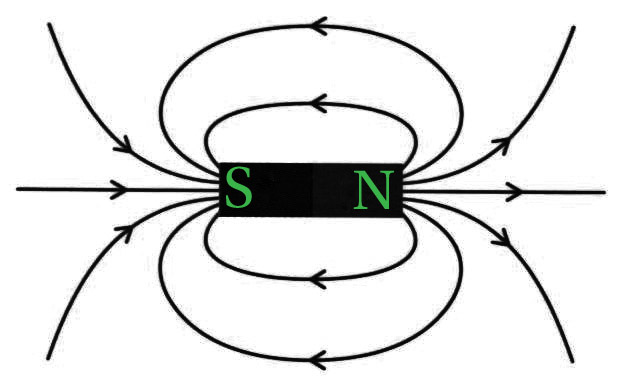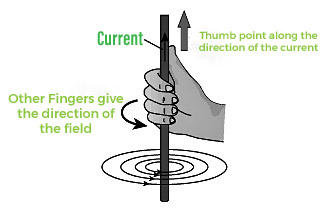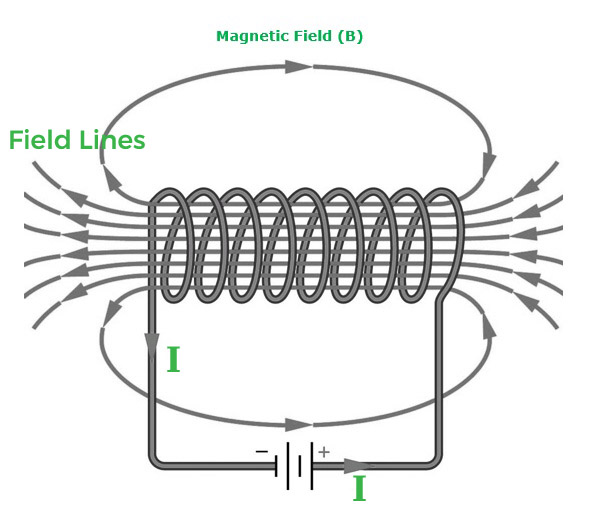# Magnetic Field due to Current carrying Conductor

• Difficulty Level : Hard
• Last Updated : 28 May, 2021

In physics, a magnet is a material that induces a magnetic field that draws or repels other magnetic materials. A magnet is always polarized, with poles called north and south, and these two poles always remain together and cannot be isolated, and when we freely suspend a magnet, the magnetic north pole will point to the geographic north of the Earth.

Magnets are found in refrigerators, radio and stereo headphones, audio and videotape players, children’s toys, and printer hard discs and floppies. Now, before we get into the magnetic field caused by a current-carrying loop and a solenoid, let’s go through some fundamental terms like a magnetic field, magnetic field lines, and solenoid as:

Attention reader! All those who say programming isn't for kids, just haven't met the right mentors yet. Join the  Demo Class for First Step to Coding Course, specifically designed for students of class 8 to 12.

The students will get to learn more about the world of programming in these free classes which will definitely help them in making a wise career choice in the future.

### Magnetic Field

A magnetic field is a force field formed by magnetic dipoles and moving electric charges that exerts a force on other surrounding moving charges and magnetic dipoles.

A magnetic field is a vector field that exists in the vicinity of a magnet, an electric current, or a shifting electric field and in which magnetic forces can be observed. Moving electric charges and inherent magnetic moments of elementary particles aligned with a fundamental quantum property known as spin generate a magnetic field.

Since it has both magnitude and direction, the magnetic field is a vector quantity.

It is denoted by symbol B

The SI unit of the magnetic field N s/C or Tesla (T).

### Magnetic Field Lines

• Magnetic field lines are imaginary lines around the magnet, and they are continuous closed loops. The tangent to the field line at any given point indicates the direction of the total magnetic field at that instant.
• Since the magnet is dipolar, the magnetic lines must be originated and also have an end. Therefore, it starts from the north pole and terminates at the south pole outside the bar magnet, and it moves from the south pole to the north pole inside the magnet.
• The closeness of field lines shows the relative strength of the magnetic field, i.e. closer lines show a stronger magnetic field and vice — versa. Crowded field lines near the poles of the magnet show more strength.Field lines of the bar magnet

### Properties of magnetic field lines

• Magnetic field boundaries are never crossed.
• The depth of the field lines shows the field’s power.
• Magnetic field lines are often closed loops.
• Magnetic field lines often originate from or begin at the north pole and end at the South Pole.

### How to find the direction of the magnetic field due to the current-carrying conductor?

When current is passed through a straight current-carrying conductor, a magnetic field is produced around it. The field lines are in the form of concentric circles at every point of the current-carrying conductor.  And we can find the direction of the magnetic field, in relation to the direction of electric current through a straight conductor can be depicted by using the Right-Hand Thumb Rule is also called as Maxwell Corkscrew Rule.Right-hand thumb rule

This rule states that ‘If a current-carrying conductor is held by the right hand, keeping the thumb straight and if the direction of electric current is in the direction of thumb, then the direction of wrapping of other fingers will show the direction of the magnetic field.’

### Magnetic field due to current through a circular loop

The right-hand thumb rule can be used for a circular conducting wire as well as it comprises small straight segments. Every point on the wire carrying current gives rise to a magnetic field around it would become larger and larger as we move away from the wire and by the time we reach the center of the circular loop, the arcs of these circle would appear as a straight line

### Magnetic field and number of turns of the coil

The magnitude of the magnetic field gets summed up with the increase in the number of turns of the coil. If there are ‘n’ turns of the coil, the magnitude of the magnetic field will be ‘n’ times of magnetic field in case of a single turn of the coil.

The strength of the magnetic field at the center of the loop (coil) depends on:

• The radius of the coil: The strength of the magnetic field is inversely proportional to the radius of the coil. If the radius increases, the magnetic strength at the center decreases
• The number of turns in the coil: As the number of turns in the coil increase, the magnetic strength at the center increases, because the current in each circular turn is having the same direction, thus, the field due to each turn adds up.
• The strength of the current flowing in the coil: As the strength of the current increases, the strength of three magnetic fields also increases.

### What is a solenoid?

The solenoid is the coil with many circular turns of insulated copper wire wrapped closely in the shape of a cylinder. A current-carrying solenoid produces a similar pattern of the magnetic field as a bar magnet. One end of the solenoid behaves as the North Pole and another end behaves as the South Pole.

### Magnetic field due to a current in a Solenoid

Magnetic field lines are parallel inside the solenoid, similar to a bar magnet, which shows that the magnetic field is the same at all points inside the solenoid. The magnetic field produced by a solenoid is similar to a bar magnet. The strength of the magnetic field is proportional to the number of turns and magnitude of the current.

By producing a strong magnetic field inside the solenoid, magnetic materials can be magnetized. A magnet formed by producing a magnetic field inside a solenoid is called an electromagnet.Field lines of solenoid

### Sample Problems

Problem 1: Explain the effect on the magnetic field produced at a point in a current-carrying circular coil due to:

(i) increase in the amount of current flowing through it,

(ii) increase in the distance of the point from the coil,

(iii) increase in the number of turns of the coil.

Solution:

(i) The magnetic field produced by current carrying circular coil is directly proportional to the current flowing through the coil. Therefore, with increase in the magnitude of magnetic field the current flowing through the coil will increase.

(ii) Magnitude of magnetic field at a point in a current carrying coil is inversely proportional to the distance. Hence, with increase in distance the magnetic field will decrease.

(iii) The magnetic field produced depends on directly to the current flowing through the circular coil. If number of turns of coil increases then the current flowing in a coil also increases and hence the magnetic field will increase with increase in number of turns.

Problem 2: How does a solenoid behave like a magnet?

Solution:

Since solenoid has iron core with insulated copper wire around it, therefore it behaves like magnet. When a current is flowing through the solenoid, magnetic field is produced around it. And the field produced is similar to the magnetic field of a bar magnet.

Problem 3: Define right-hand thumb rule?

Solution:

This rule states that ‘If a current carrying conductor is held by right hand, keeping the thumb straight and if the direction of electric current is in the direction of thumb, then the direction of wrapping of other fingers will show the direction of magnetic field.’

Problem 4: Why don’t two magnetic field lines cannot intersect each other?

Solution:

All Field lines follow their own path to reach from the North Pole to the South Pole. Two magnetic field lines do not intersect each other because if there was point of intersection, then there would be two tangents for a single point which means that the magnetic field has two directions, which is not possible.

Problem 5: What are magnetic field lines? List two characteristic properties of these lines.

Solution:

The lines drawn around the magnetic field of any magnet is known as magnetic field lines which are also be used to determine the direction of the magnetic field.

Properties of magnetic lines of force:

• Outside the magnet the field lines originates from north pole and ends at the South Pole.
• The magnetic field lines are continuous closed loop.

My Personal Notes arrow_drop_up